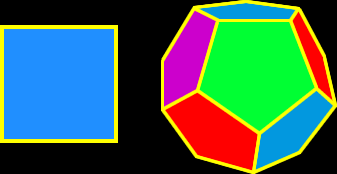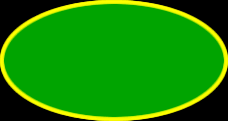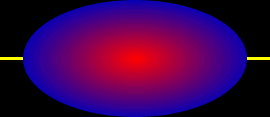# Online Math Dictionary: E

Easy to understand math definitions for K-Algebra mathematics
Just scroll down or click on the word you want and I'll scroll down for you!

 edge ellipse ellipsoid empty set equality equation equidistant equilateral triangle estimate even number expanded notation exponent exponential exterior angle

 EdgeThe edges of a polygon are the outside boundaries.  In thesquare on the right, you can see four  edges.   In a polyhedron, like the dodecahedron on the right, an edge is where two faces meet.  The edges are the yellow lines.EllipseThere's an easy definition for an ellipse and a harder algebra definition.  Here's the easy one:   An ellipse is a stretched out circle. For the algebra definition, check out.Coolmath Algebra EllipsesEllipsoidAn ellipsoid is a three dimensional ellipse.  Imagine putting a line through the longest part of an ellipse and spinning it.  This would give you an ellipsoid.Empty SetAn empty set is the same as a "null set."  An empty set is a set with no members (or elements.)  The symbol for the empty set is on the right.  It can also be written as an empty set of set brackets:                                         {   } Putting the empty set symbol inside the set brackets is a bad thing...  But, is a common mistake, so don't do it!